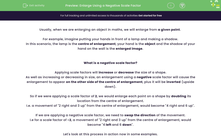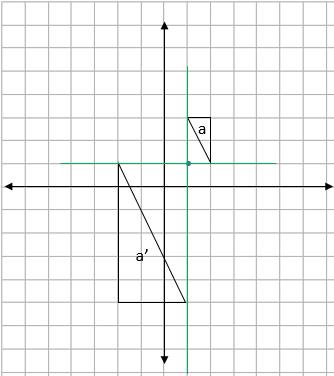# Enlarge Using a Negative Scale Factor

In this worksheet, students will enlarge shapes from a given point by applying a negative scale factor, as well as find the centre of enlargement and scale factor after an enlargement has occurred.Key stage:  KS 4

Year:  GCSE

GCSE Subjects:   Maths

GCSE Boards:   AQA, Eduqas, Pearson Edexcel, OCR,

Curriculum topic:   Geometry and Measures, Congruence and Similarity

Curriculum subtopic:   Properties and Constructions Plane Isometric Transformations

Popular topics:   Geometry worksheets

Difficulty level:#### Worksheet Overview

Usually, when we are enlarging an object in maths, we will enlarge from a given point

For example, imagine putting your hands in front of a lamp and making a shadow.

In this scenario, the lamp is the centre of enlargement, your hand is the object and the shadow of your hand on the wall is the enlarged image.

What is a negative scale factor?

Applying scale factors will increase or decrease the size of a shape.

As well as increasing or decreasing in size, an enlargement using a negative scale factor will cause the enlargement to appear on the other side of the centre of enlargement, plus it will be inverted (upside down).

So if we were applying a scale factor of 2, we would enlarge each point on a shape by doubling its location from the centre of enlargement.

I.e. a movement of "2 right and 3 up" from the centre of enlargement, would become "4 right and 6 up".

If we are applying a negative scale factor, we need to swap the direction of the movement.

I.e for a scale factor of -2, a movement of "2 right and 3 up" from the centre of enlargement, would become "4 left and 6 down".

Let's look at this process in action now in some examples.

e.g. Enlarge the shape shown below by a scale factor of -2 about the point (1,0).

Step 1: Draw the centre of enlargement on the grid, so we won't forget where we are enlarging from:Step 2: Find the movement from the centre of enlargement to one of the points on the shape:We can see that to get to the bottom left corner, we need to move 1 square right and 2 up.

Step 3: Multiply this movement by the scale factor:

Our scale factor is -2, so this movement doubles and changes direction to become 2 squares left and 4 down.

Step 4: Plot the first point on the diagram (when we are doing this, it is essential to count from the specified centre of enlargement not (0,0)):Step 5: Repeat steps 2 - 4 for the other corners of the shape:Step 6: Draw the enlarged shape and label the image and enlargement:e.g. Shape a has been enlarged to become shape a'. Find the scale factor and centre of enlargement.Finding the scale factor

We need to look closely at one of the pairs of sides.

If we use the horizontal base of each triangle, shape a is 1 square long and shape a' is 3 squares long.

As the centre of enlargement is between the two shapes and the shape has turned around during the process of enlargement, we know that we must have a negative scale factor.

This means that our scale factor is -3.

Finding the centre of enlargement

To find this, we need to join up two pairs of matching corners with straight lines and see where they cross.

We need to be really careful to join the matching pairs of corners, remembering that the shape has flipped upside down:We can see here that the two lines cross at the point (1,1), so this is our centre of enlargement.

In this activity, we will enlarge shapes from a given point by applying a negative scale factor, as well as find the centre of enlargement and scale factor after an enlargement has occurred.

You may find it helpful to have a pencil and some squared paper handy to support you in this activity. If you do not, you will need to be able to visualise these enlargements in your mind's eye.

### What is EdPlace?

We're your National Curriculum aligned online education content provider helping each child succeed in English, maths and science from year 1 to GCSE. With an EdPlace account you’ll be able to track and measure progress, helping each child achieve their best. We build confidence and attainment by personalising each child’s learning at a level that suits them.

Get started